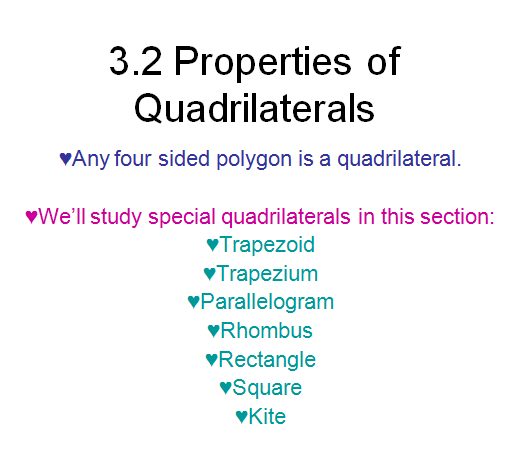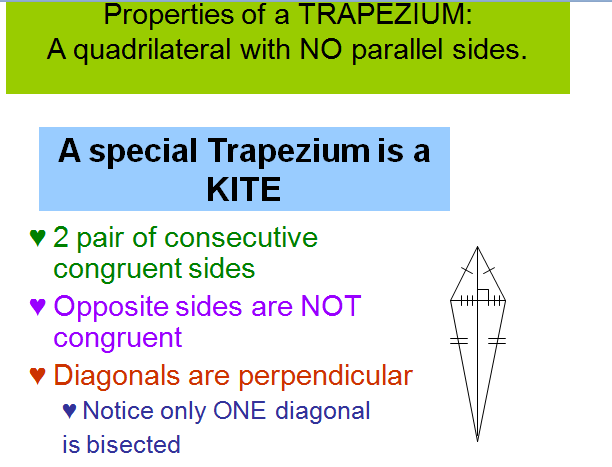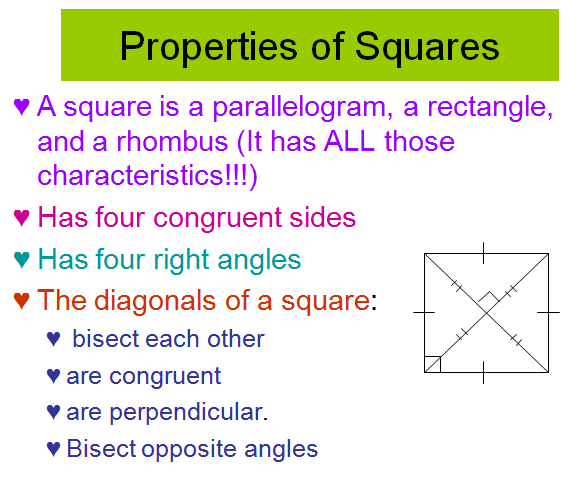# SCC Education

## Properties of quadrilaterals ,trapezoid ,trapezium,parallelogram,rhombus,rectangle,square ,kite,Mathematics for class 8 ..........

Formula-sheet
It will help you to solve the questions of mensuration like area ,volume ,Surface area etc.

Rule of divisibility

It will help you to under stand rule of divide .how to check a number is divisible or not.

A fraction is known as a rational number and written in the form of p/q where p and q are  integers and q ≠ 0. The lower number ‘q’ is known as denominator and the upper  number ‘p’ is known as numerator. Type of Fractions Proper Fraction: The fraction in which numerator is less the denominator is called a  proper fraction.

Angles An angle is formed when two lines meet. The size of the angle measures the amount of space between the lines. In the diagram the lines ba and bc are called the ‘arms’ of the angle, and the point ‘b’ at which they meet is called the ‘vertex’ of the angle.

Properties-of-parallel-lines

Parallelograms properties

Volume-and-area-of-cone-and-pyramid

Profit-and-loss-questions

Compound-interest

Volumes-surface-and-area

Percentage-and-its-application

Test paper Algebra

Test paper redicals

Rational number

Profit and loss

Work problem

Power questions

formula-sheet

properties-of-parallel-lines

parallelograms properties

volume-and-area-of-cone-and-pyramid

profit-and-loss-questions

compound-interest

volumes-surface-and-area

percentage-and-its-application